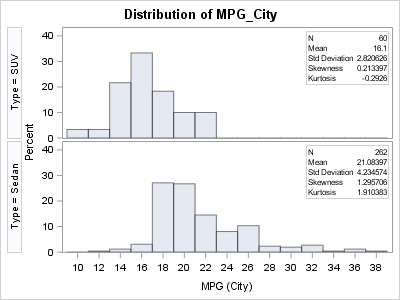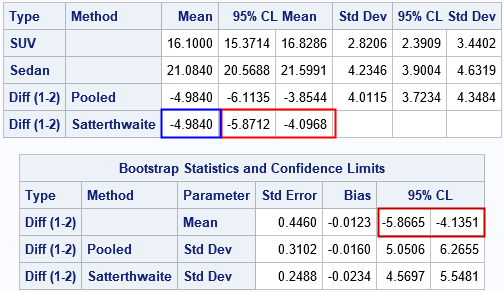Bootstrap resampling is a powerful way to estimate the standard error for a statistic without making any parametric assumptions about its sampling distribution. The bootstrap method is often implemented by using a sequence of calls to resample from the data, compute a statistic on each sample, and analyze the bootstrap distribution. An example is provided in the article "Compute a bootstrap confidence interval in SAS." This process can be lengthy and in Base SAS it requires reading and writing a large amount of data. In SAS/STAT 14.3 (SAS 9.4m5), the TTEST procedure supports the BOOTSTRAP statement, which automatically performs a bootstrap analysis of one-sample and two-sample t tests. The BOOTSTRAP statement also applies to two-sample paired tests.

### The difference of means between two groups

The BOOTSTRAP statement makes it easy to obtain bootstrap estimates of bias and standard error for a statistic and confidence intervals (CIs) for the underlying parameter. The BOOTSTRAP statement supports several estimates for the confidence intervals, including normal-based intervals, t-based intervals, percentile intervals, and bias-adjusted intervals. This section shows how to obtain bootstrap estimates for a two-sample t test. The statistic of interest is the difference between the means of two groups.

The following SAS DATA step subsets the Sashelp.Cars data to create a data set that contains only two types of vehicles: sedans and SUVs. A call to PROC UNIVARIATE displays a comparative histogram that shows the distributions of the MPG_City variable for each group. The MPG_City variable measures the fuel efficiency (in miles per gallon) for each vehicle during typical city driving.

```/* create data set that has two categories: 'Sedan' and 'SUV' */ data Sample; set Sashelp.Cars(keep=Type MPG_City); if Type in ('Sedan' 'SUV'); run;   proc univariate data=Sample; class Type; histogram MPG_City; inset N Mean Std Skew Kurtosis / position=NE; ods select histogram; run;```### Bootstrap estimates for a two-sample t test

Suppose that you want to test whether the mean MPG of the "SUV" group is significantly different from the mean of the "Sedan" group. The groups appear to have different variances, so you would probably choose the Satterthwaite version of the t test, which accommodates different variances. You can use PROC TTEST to run a two-sample t test for these data, but in looking at the distributions of the groups, you might be concerned that the normality assumptions for the t test are not satisfied by these data. Notice that the distribution of the MPG_City variable for the "Sedan" group has high skewness (1.3) and moderately high kurtosis (1.9). Although the t test is somewhat robust to the normality assumption, you might want to use the bootstrap method to estimate the standard error and confidence interval for the difference of means between the two groups.

If you are using SAS/STAT 14.3, you can compute bootstrap estimates for a t test by using the BOOTSTRAP statement, as follows:

```title "Bootstrap Estimates with Percentile CI"; proc ttest data=Sample; class Type; var MPG_City; bootstrap / seed=123 nsamples=10000 bootci=percentile; /* or BOOTCI=BC */ run;```The BOOTSTRAP statement supports three options:

• The SEED= option initializes the internal random number generator for the TTEST procedure.
• The NSAMPLES= option specifies the number of bootstrap resamples to be drawn from the data.
• The BOOTCI= option specifies the estimate for the confidence interval for the parameter. This example uses the PERCENTILE method, which uses the α/2 and 1 – α/2 quantiles of the bootstrap distribution as the endpoints of the confidence interval. A more sophisticated second-order method is the bias-corrected interval, which you can specify by using the BOOTCI=BC option. For educational purposes, you might want to compare these nonparametric estimates with more traditional estimates such as t-based confidence intervals (BOOTCI=TBOOTSE).

The TTEST procedure produces several tables and graphs, but I have highlighted a few statistics in two tables. The top table is the "ConfLimits" table, which is based on the data and shows the traditional statistics for the t test. The estimate for the difference in means between the "SUV" and "Sedan" groups is -4.98 and is highlighted in blue. The traditional (parametric) estimate for a 95% confidence interval is highlighted in red. The interval is [-5.87, -4.10], which does not contain 0, therefore you can conclude that the group means are significantly different at the 0.05 significance level.

The lower table is the "Bootstrap" table, which is based on the bootstrap resamples. The TTEST documentation explains the resampling process and the computation of the bootstrap statistics. The top row of the table shows estimates for the difference of means. The bootstrap estimate for the standard error is 0.45. The estimate of bias (which subtracts the average bootstrap statistic from the sample statistic) is -0.01, which is small. The percentile estimate for the confidence interval is [-5.87, -4.14], which is similar to the parametric interval estimate in the top table. (For comparison, the bias-adjusted CI is also similar: [-5.85, -4.12].) Every cell in this table will change if you change the SEED= or NSAMPLES= options because the values in this table are based on the bootstrap samples.

Although the difference of means is the most frequent statistic to bootstrap, you can see from the lower table that the BOOTSTRAP statement also estimates the standard error, bias, and confidence interval for the standard deviation of the difference. Although this article focuses on the two-sample t test, the BOOTSTRAP statement also applies to one sample t tests.

In summary, the BOOTSTRAP statement in PROC TTEST in SAS/STAT 14.3 makes it easy to obtain bootstrap estimates for statistics in one-sample or two-sample t tests (and paired t tests). By using the BOOTSTRAP statement, the manual three-step bootstrap process (resample, compute statistics, and summarize) is reduced to a zero-step process. The TTEST procedure handles the details for you. Of course, if you are using an earlier release of SAS software, you can always implement the bootstrap method for t tests manually.

Share